# Central Park

Central Park is a rectangular park in New York City and the map scale is 1 cm: 320 m (It means 1 cm on the map represents 320 m in the real world. ). Find the north-south distance if the length on the map is 2.5 cm.

x =  800 m

### Step-by-step explanation: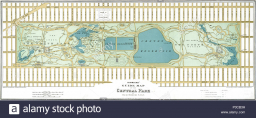Did you find an error or inaccuracy? Feel free to write us. Thank you!Tips to related online calculators
Do you want to convert length units?

## Related math problems and questions:

• A map 3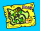A map is drawn to a scale of 1 : 25 000. This scale can be expressed as 1 cm represents n km. Find n.
• Two citiesOn a map, 1.5 cm represents 50 miles. If the distance between the two cities on the map is 6 cm, how many miles separate the cities?
• Two villages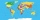Two villages are 11 km and 500 m away. On the map, their distance is determined by a 5 cm long line. Find the scale of the map.
• Map scaleGarden has on plan on a scale of 1: 150 width 22 cm and length 35 cm. What is the real area of the garden?
• The scaleThe distance of 15 cm on the map corresponds to a distance of 27 km in reality. What is the scale of the map?
• Nautical miles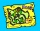How many nautical miles do they sail if the route is shown on a 1:25 000 scale map with a 7.4 cm long line?
• Scale of the mapDetermine the scale of the map if the actual distance between A and B is 720 km and the distance on the map is 20 cm.
• On the mapA line 1.5 cm long corresponds to a line 3 cm long on the map. What is the scale of the map?
• Map 2At what scale is made map if the distance 8.2 km corresponds on the map segment 5 cm long?
• Hectares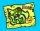Determine the area of the rectangular land (in ha), which has dimensions of 3 cm and 4.5 cm on the plan with a scale of 1: 40,000.
• Scale 3Miriam room is 3.2 meters wide. It is draw by line segment length 6.4 cm on floor plan. In what scale it is plan of the room?
• The distance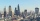The distance between two towns on a given map is 3 ½ cm. If ½ cm represents 6km. , what is the distance between the two towns?
• The plan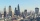The plan of the housing estate is in three scales 1: 5000,1: 10000,1: 15000. The distance between two points on a plan with a scale of 1: 10000 is 12 cm. What is this distance on the other two plans? What is this distance?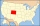The state Colorado has the shape of a rectangle. The map is sold on 2 posters. The first has dimensions of 70 cm and 50 cm in a scale of 1: 1,000,000. The second poster has dimensions of 175 cm and 125 cm. What is the scale of the second poster?On a 1:1000 scale map is a rectangular land of 4.2 cm and 5.8 cm. What is the area of this land in square meters?On the plan of the village in the scale of 1: 1000 a rectangular garden is drawn. Its dimensions on the plan are 25mm and 28mm. Determine the area of the garden in ares.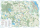A map represents every 4 miles with 1 inch. If a school and a bank are actually 12 miles apart, how far apart are they on the map, in inches?